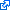﻿ Features of Matrix eQTL

## I see systematic inflation of test statistics in QQ-plot. What are the possible causes and how to fix that?

The linear regression model used by Matrix eQTL is built on a set of assumptions about the data generation process and is susceptible to violations of these assumptions. The important assumptions are:

• Normal distribution of the errors. Lack of outliers.
• Errors are uncorrelated and have the same variance.

### • Outliers in genotype. Minor allele frequency filtering.

SNPs with low minor allele frequency are non-informative (experience too little variation) and have a great potential for creating spurious findings. It is a common practice to filter out such SNPs. It can be done within code running Matrix eQTL:

`maf.list = vector('list', length(snps))for(sl in 1:length(snps)) {  slice = snps[[sl]];  maf.list[[sl]] = rowMeans(slice,na.rm=TRUE)/2;  maf.list[[sl]] = pmin(maf.list[[sl]],1-maf.list[[sl]]);}maf = unlist(maf.list)## Look at the distribution of MAF# hist(maf[maf<0.1],seq(0,0.1,length.out=100))cat('SNPs before filtering:',nrow(snps))# snps\$RowReorderSimple(maf>0.05);snps\$RowReorder(maf>0.05);cat('SNPs before filtering:',nrow(snps))`

### • Outliers in expression. Quantile normalization and Kruskal-Wallis test.

Outliers in expression data are usually harder to deal with. The accepted remedy by the GTEx consortium is the transformation of the measurements for each gene into normally distributed while preserving relative rankings. The target distribution may be the standard normal distribution or the normal distribution the mean and spread of the original measurements. Here is the code for such transformation:

`for( sl in 1:length(gene) ) {  mat = gene[[sl]];  mat = t(apply(mat, 1, rank, ties.method = "average"));  mat = qnorm(mat / (ncol(gene)+1));  gene[[sl]] = mat;}rm(sl, mat);`

A similar approach allows one to run Kruskal-Wallis testswith Matrix eQTL. In this case one has to use the ANOVA model (`useModel = modelANOVA`) and transform the measurements for each gene into their respective ranks.

`for( sl in 1:length(gene) ) {  mat = gene[[sl]];  mat = t(apply(mat, 1, rank, ties.method = "average"));  gene[[sl]] = mat;}rm(sl, mat);`

## Matrix eQTL does not report [X]. How can I get that?

Matrix eQTL is designed for very fast testing of millions of hypotheses using linear regression model. It reports only essential statistics and only for those tests that pass significance threshold. Right now Matrix eQTL can report the following information.

• For tests that pass significance threshold:
• statistic: Test statistic (t-statistic of T-test)
• beta: Effect size estimate
• p-value: p-value, as would be calculated by any linear regression software.
• FDR: False discovery rate estimated with Benjamini–Hochberg procedure• Distribution of all p-values, including those not passing the significance threshold
(see `pvalue.hist` parameter of `Matrix_eQTL_main`).
• Best p-value for each gene and each SNP, including those not passing the significance threshold
(see `min.pv.by.genesnp` parameter of `Matrix_eQTL_main`).

### • Standard errors for the estimates.

Matrix eQTL currently reports estimates of the effect size (slope coefficient) and the corresponding t-statistics, but does not report the standard errors for the effect size. By definition a t-statistic is equal to the effect size estimate divided by its standard error. Thus, the standard deviation of the estimate can be easily calculated as the ration of the effect size estimates and their t-statistics.

`me\$all\$eqtls\$beta_se = me\$all\$eqtls\$beta / me\$all\$eqtls\$statistic;`

### • Raw correlations from which Matrix eQTL calculates t-statistics and p-values.

First, you would need to know the number of degrees of freedom for the full model estimated by Matrix eQTL. It is recorded in the object returned by Matrix eQTL main function:

`dfFull = me\$param\$dfFull;`

To get the correlations from t-statistics apply the following formula:

`tstat = me\$cis\$eqtls\$statistic;`
`r = tstat / sqrt( dfFull + tstat^2 );`

To get R-squared for each gene-SNP pair just square the correlation:

`R2 = r^2;`

### • Residuals, significance of covariates, etc...

Matrix eQTL gains its high performance by avoiding calculations of anything non-essential. In particular, Matrix eQTL does neither calculate nor, naturally, report the residuals or the significance and effect sizes of the covariates. To obtain these values one would have to run linear regression on selected gene-SNP pairs manually using R or other tools.

By Andrey Shabalin